# Titration of a Weak Base Prelaboratory Questions 1. Will addition of HCl to a solution make...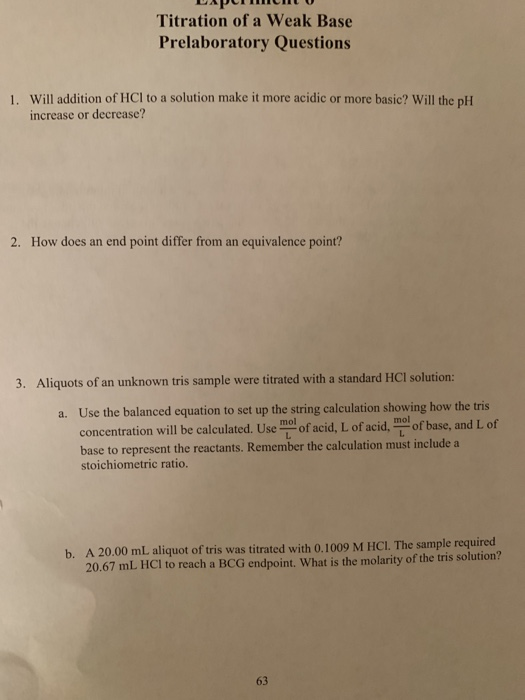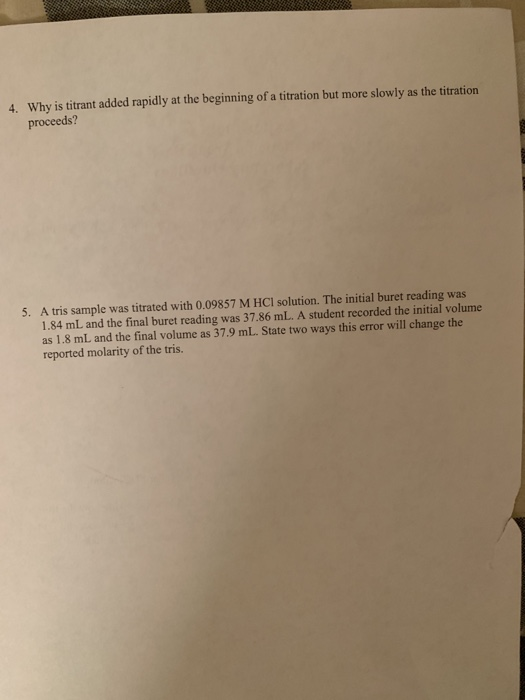Titration of a Weak Base Prelaboratory Questions 1. Will addition of HCl to a solution make it more acidic or more basic? Will the pH increase or decrease? How does an end point differ from an equivalence point? 2. were titrated with a standard HCl solution: 3. Aliquots of an unknown tris sample Use the balanced equation to set up the string calculation showing how the tris a. mol of acid, L of acid. mo of base, and L of concentration will be calculated. Use L base to represent the reactants. Remember the calculation must include a stoichiometric ratio. b. A 20.00 mL aliquot of tris was titrated with 0.1009 M HCI. The sample required 20.67 mL HCI to reach a BCG endpoint. What is the molarity of the tris solution? 63
4. Why is titrant added rapidly at the beginning of a titration but more proceeds? slowly as the titration 5. A tris sample was titrated with 0.09857 M HCl solution. The initial buret reading was 1.84 mL and the final buret reading was 37.86 mL. A student recorded the initial volume as 1.8 mL and the final volume as 37.9 mL. State twoways this error will change the reported molarity of the tris.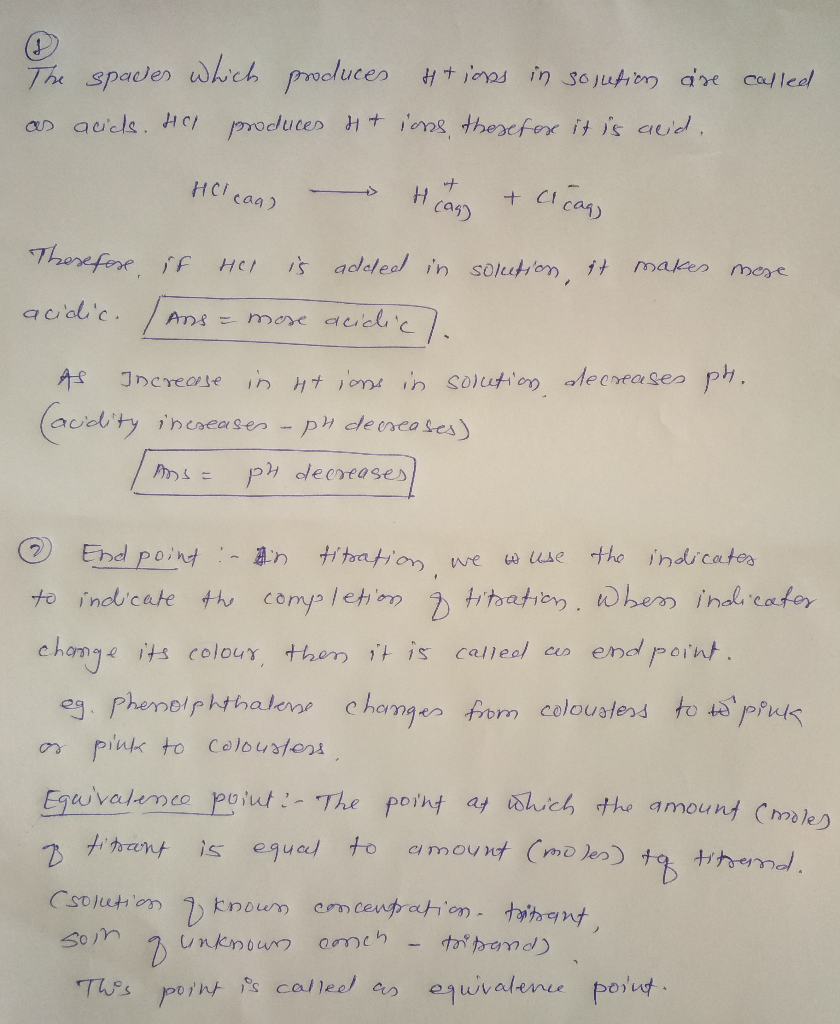#### Earn Coin

Coins can be redeemed for fabulous gifts.

Similar Homework Help Questions
• ### A titration of 25.00 mL of O.1550 M HCl solution with a solution of NaOH of...

A titration of 25.00 mL of O.1550 M HCl solution with a solution of NaOH of unknown molarity starts at a buret reading for NaOH of 0.33 mL The phenolphthalein indicator turns light pink the acid solution for over 30 seconds at a buret reading of 24.19 mL 2) What was the volume of HCl you started with? 3) How many moles of HCl were in the original solution? 4) Write the balanced chemical equation for the titration reaction. 5)...

• ### can you help with the balance equation and also the calculations at the bottom of the...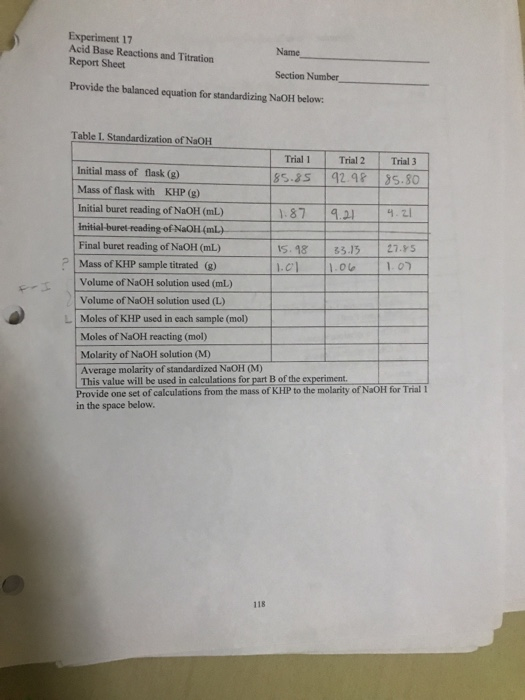can you help with the balance equation and also the calculations at the bottom of the sheet thank you Experiment 17 Acid Base Reactions and Titration Report Sheet Name Section Number Provide the balanced equation for standardizing NaOH below. Table L. Standardization of NaOH Trial Trial 2 Trial 3 Initial mass of flask (g) 85.85 92.9285.80 Mass of flask with KHP (g) Initial buret reading of NaOH (mL) 11.87 19.21 4.21 Initial-buret-reading of NaOH (mL) Final buret reading of NaOH...

• ### Data Standard solution: NaOH concentration 0.25 M Volume of HCl used (Va) Initial NaOH buret reading...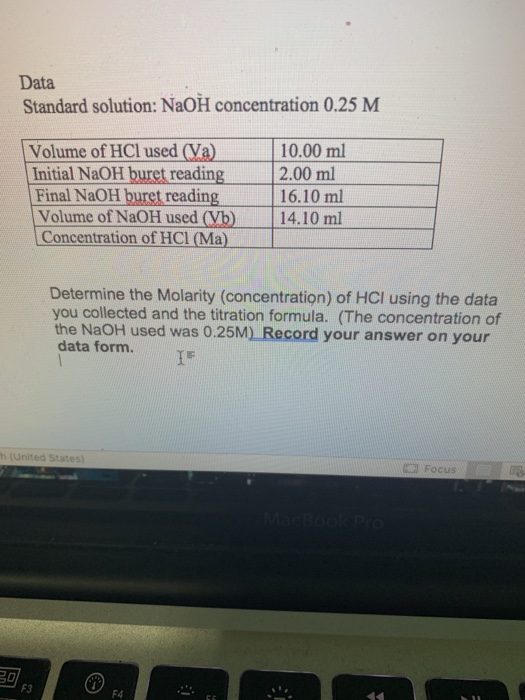Data Standard solution: NaOH concentration 0.25 M Volume of HCl used (Va) Initial NaOH buret reading Final NaOH buret reading Volume of NaOH used (Vb) Concentration of HCl (Ma) 10.00 ml 2.00 ml 16.10 ml 14.10 ml Determine the Molarity (concentration) of HCI using the data you collected and the titration formula. (The concentration of the NaOH used was 0.25M) Record your answer on your data form. United States Focus 17. It takes 75ml of a 2.5M HCl solution to...

• ### Determine how many liters (l) of stock HCl were used in titration #1. Please fill all...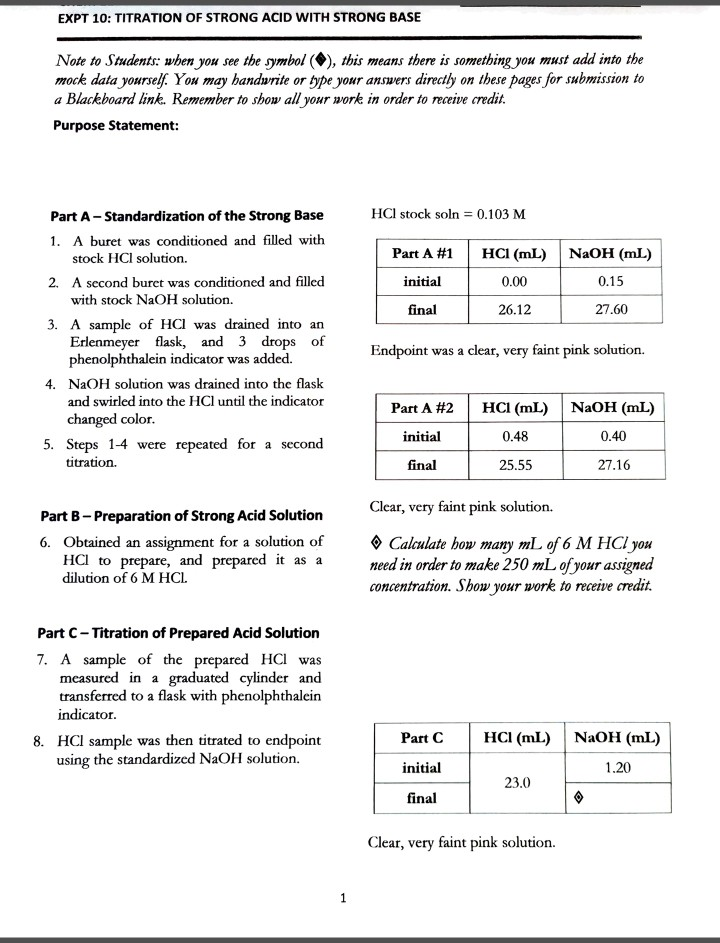Determine how many liters (l) of stock HCl were used in titration #1. Please fill all questions under "Data Analysis" Page 1 is just instruction for Page 2 EXPT 10: TITRATION OF STRONG ACID WITH STRONG BASE Note to Students: when you see the symbol (), this means there is something you must add into the mock data yourself. You may handwrite or type your answers directly on these pages for submission to a Blackboard link. Remember to show all...

• ### Date: Thermodynamics Data Part 1 Temperature of Ca(OH)2 solution: 20°C Molarity of the HCl solution used...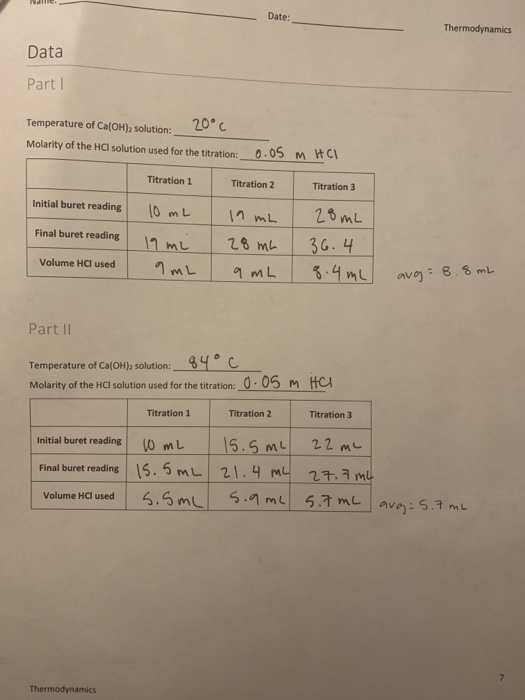Date: Thermodynamics Data Part 1 Temperature of Ca(OH)2 solution: 20°C Molarity of the HCl solution used for the titration: 0.05 M H CH Titration 1 Titration 2 Titration 3 Initial buret reading Final buret reading 10 mL 19 mi 9mL 11 mL 20 ml | 28 mc 36.4 9 mL q.4mL Volume HCl used avg=8.8 mL Part II Temperature of Ca(OH)solution: 84 c Molarity of the HCl solution used for the titration: 0.05 M HCI Titration 1 Titration 2 Titration...

• ### Standardization of a NaOH Solution and Subsequent Titration of a HCl Solution of Unknown Molarity Report...Standardization of a NaOH Solution and Subsequent Titration of a HCl Solution of Unknown Molarity Report Sheets Data Table 1: Standardization of NaOH Trial 1 Trial 2 Trial 3 Mass KHP 1.00 1.009 11.00g Initial volume in buret (ml) 41.000 ML 35.800 mL 30.600ml Final volume in buret (ML) 35.900ML er 30.6c0ml 25.400ml Volume of NaOH used (ml) 52.000 52.000 52.000 Volume of NaOH (L) 156.1940 mL 59.99 L sl. glemt 51.91ml Moles KHP titrated Moles NaOH used Molarity of...

• ### Experiment Lab Team Members Finding Equilibrium Constants: The Solubility of Borax Report Sheet Part A: Titration...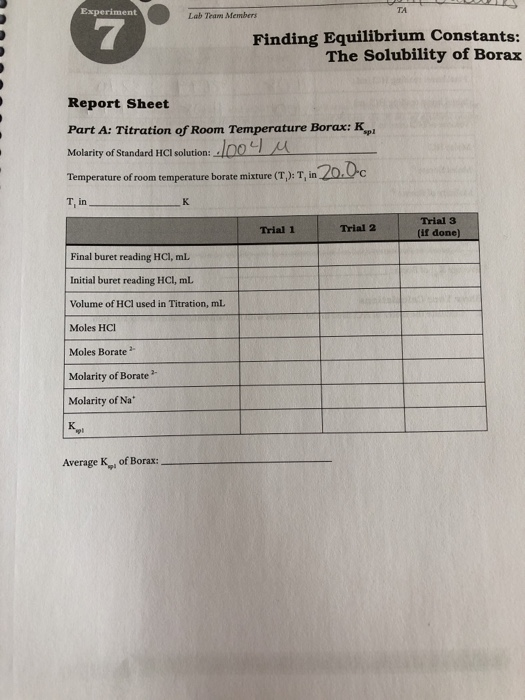Experiment Lab Team Members Finding Equilibrium Constants: The Solubility of Borax Report Sheet Part A: Titration of Room Temperature Borax: K Molarity of Standard HCl solution: 004 u Temperature of room temperature borate mixture (T): T, in 20.0c T, in к Trial Trial 2 Trial 3 if donel Final buret reading HCI, ml. Initial buret reading HCl, mL Volume of HCl used in Titration, ml. Moles HCI Moles Borate Molarity of Borate Molarity of Na Average of Borax: Part B:...

• ### help;( Data Sheet Titration and Buffers Name Date Part One: Buffer Solution (record the results from...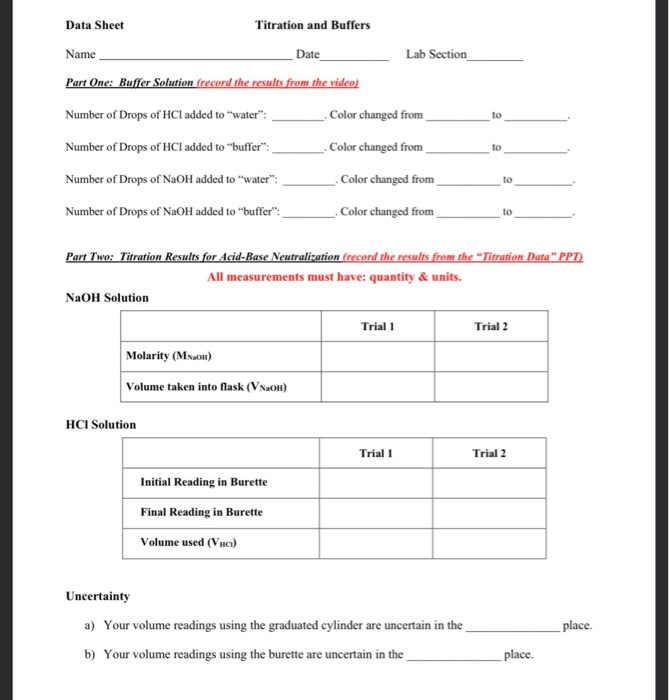help;( Data Sheet Titration and Buffers Name Date Part One: Buffer Solution (record the results from the video) Lab Section Number of Drops of HCl added to "water": Color changed from to Number of Drops of HCl added to "buffer": Color changed from to to Number of Drops of NaOH added to "water": Number of Drops of NaOH added to "buffer": _Color changed from Color changed from to Part Two: Titration Results for Acid-Base Neutralization (record the results from the...

• ### Standardization of NaOH: Acid Base Titration Objective: In this lab, you will accurately determine the concentration...

Standardization of NaOH: Acid Base Titration Objective: In this lab, you will accurately determine the concentration of a solution of sodium hydroxide (NaOH) using a 0.500M potassium hydrogen phthalate (KHP) standard solution. Background: Acid–Base Titrations When an acid reacts with a base, a neutralization reaction occurs. The H+ ions from the acid and the HO– ions from the base combine to form water and are therefore neutralized. The other product of reaction is a salt. For example, hydrochloric acid reacts...

• ### 1. What is the definition of an 'equivalence point' in an acid/base titration? (1 point) 2....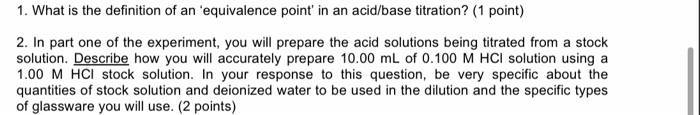1. What is the definition of an 'equivalence point' in an acid/base titration? (1 point) 2. In part one of the experiment, you will prepare the acid solutions being titrated from a stock solution. Describe how you will accurately prepare 10.00 mL of 0.100 M HCl solution using a 1.00 M HCl stock solution. In your response to this question, be very specific about the quantities of stock solution and deionized water to be used in the dilution and the...

Free Homework App